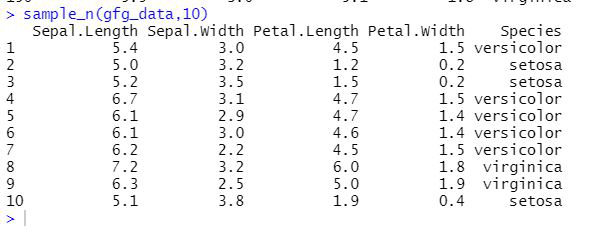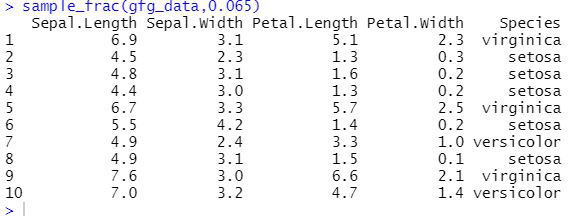Related Articles

# Select Random Samples in R using Dplyr

• Last Updated : 28 Jul, 2021

In this article, we will be looking at different methods for selecting random samples from the Dplyr package of the R programming language.

To install and import the Dplyr package in the R programming language, the user needs to follow the syntax:

Syntax:

install.packages(“dplyr”)

library(dplyr)

## Method 1: Using Sample_n() function

Sample_n() function is used to select n random rows from a dataframe in R. This is one of the widely used functions of the R programming language as this function is used to test the various user build models for prediction and for accuracy purposes.

Syntax: sample_n(tbl, size, replace, fac, …)

Parameters:

• tbl: a Momocs object (Coo, Coe)
• size: numeric how many shapes should we sample
• replace: logical whether the sample should be done with or without replacement
• fac: a column name if a \$fac is defined; size is then applied within levels of this factor
• …: additional arguments to dplyr::sample_n and to maintain generic compatibility

Return:

This function will be returning the random samples from the provided data of size n.

Example: R program to generate random sample using sample_n()

## R

 `library``(dplyr)`` ` `gfg_data <- iris``sample_n``(gfg_data,10)`

Output:## Method 2: Using Sample_frac() function

Sample_frac() function selects a random n percentage of rows from a dataframe or table, the use of this function is similar to the sample_n() function, and this function is widely used in the R programming language.

Syntax: sample_frac(tbl, size, replace, fac, …)

Parameters:

• tbl: a Momocs object (Coo, Coe)
• size: numeric (0 < numeric <= 1) the fraction of shapes to select
• replace: logical whether the sample should be done with or without replacement
• fac: a column name if a \$fac is defined; size is then applied within levels of this factor
• …: additional arguments to dplyr::sample_frac and to maintain generic compatibility

Example: R program to generate random sample using sample_frac()

## R

 `library``(dplyr)`` ` `gfg_data <- iris``sample_frac``(gfg_data,0.065)`

Output:My Personal Notes arrow_drop_up